# What is a Hydraulic Press? How do Hydraulic Press Works?

## What are Hydraulic Presses Used For?

Based on the nature of work, a hydraulic press may be modified to suit the need.

Hydraulic press used in industries are generally converted or built to crush or to press any “process” or the “product". For example, this may include steel plates, aluminum rolls, metallic ores, etc. In this article, we will discuss the general priciple of hydraulic presses followed by the details of the actual industrial hydraulic press.

## What are the Components of a simple Hydraulic Press?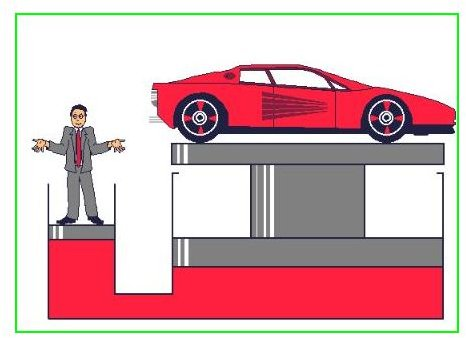The system is a very simple example which demonstrates the operation of a basic hydraulic system. It has two simple cylinders connected to each other, containing an adequate quantity of hydraulic fluid in it. One of the cylinders is larger in size when compared to the other. Both the cylinders have pistons in them, but in strict “hydraulic” terminology, the larger piston is called as a “ram” and small one a “plunger”. As seen from the figure, a small force “P” applied on the plunger, in the downward direction, presses the hydraulic fluid below it. This pressure is transmitted equally in all directions and raises the “ram” (Pascal’s Law). The heavier load placed on the “ram” is thus lifted up.

## Wondering how a small force can lift up a heavy load?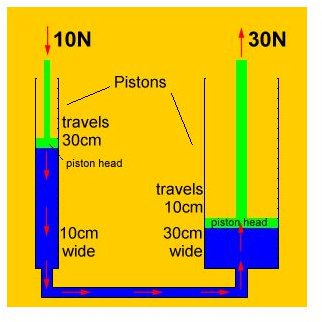The force applied on the plunger may be small when compared to the weight placed on the ram. Also, the area of the plunger is small when compared to area of the ram. But the pressure acting on the plunger (due to the application of force “F” on the plunger), and the ram is same (Pascal’s Law). It is the area on which the pressure is acting that makes the difference. The pressure “P” acts on the ram, which has a large area. The same pressure “P” acting on the plunger has a small area. Also the distance traveled by the plunger is more when compared to the distance traveled by the ram. This makes a small force applied on the plunger able to lift heavy loads placed on the ram.

## Mechanical Advantage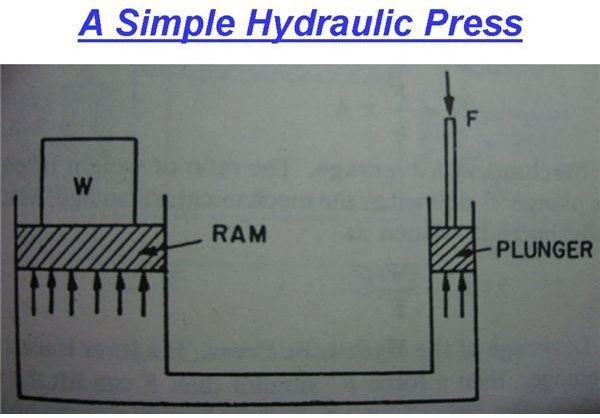Let W = weight to be lifted,

F = force applied on the plunger,

A = area of the ram,

“a” = area of the plunger,

P = pressure intensity produced by force F.

We know that pressure P = Force / Area of plunger

P = F/a

As per the Pascal’s Law, this pressure P, due to the force acting on the plunger, is equally acting on all parts of the hydraulic system. So this same pressure acts on the ram too. So

P = F/a

But considering only the ram,

Pressure P = W/A.

So equating both the pressures, as they have to be equal,

W/A = F/a

So W = A/a * F.

But mechanical advantage can be defined as “the ratio of weight lifted to the force acting on the plunger.”

So M.A = W/F.

## Leverage for a Hydraulic Press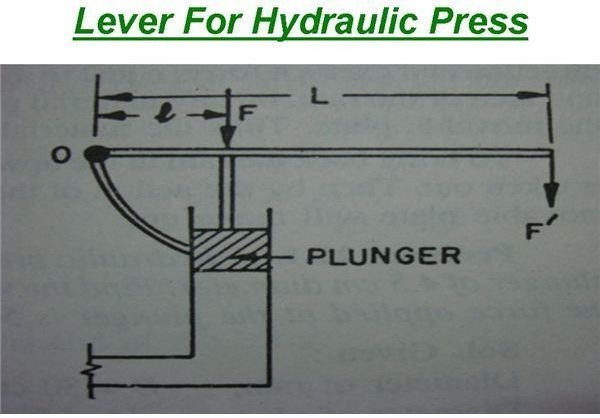A lever is used to gain mechanical advantage when lifting heavy weights for which hydraulic systems already have reduced the force which has to be applied by multiples. By using a lever in combination with a hydraulic press, the mechanical advantage gained is further optimized.

## Actual Heavy Hydraulic Press: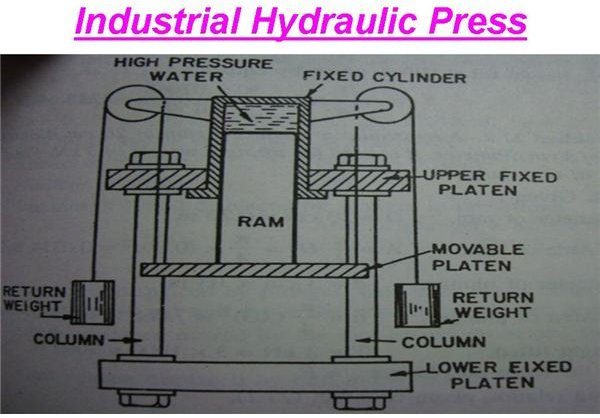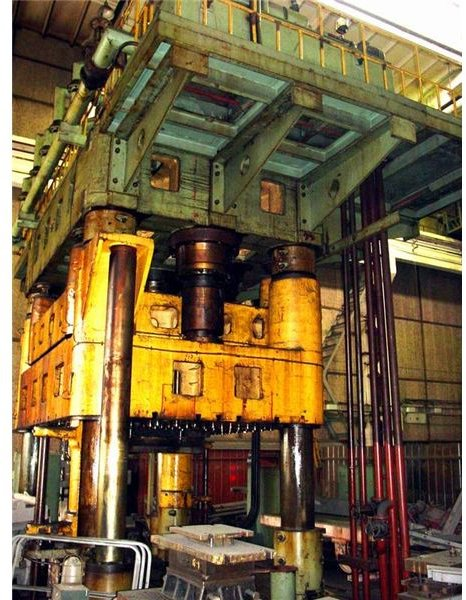The picture at the left represents a typical industrial hydraulic press. It has a fixed cylinder in which a ram is sliding. To the lower end of the ram, a movable plate is attached. As the ram moves up and down, the movable plate attached to the ram also moves up and down between two fixed plates. When a hydraulic fluid is supplied under high pressure into the cylinder, the ram moves down exerting a force, which is equal to the product of the intensity of the pressure of hydraulic fluid and the area of the ram on which it acts. Thus any material placed between the movable plate and the lower fixed plate gets pressed or even crushed depending on the pressure of the oil supplied. The return weights, which are suspended along with the movable plate, ensure that the ram moves back up after the pressure inside the cylinder is released.

Thus we have seen the working of the hydraulic press and in my next article, we will discuss hydraulic accumulators.

References:

www.tpub.com

www.alibaba.com

https://leo.koppel.ca/backhoe/hydraulics.html

A Text Book Of Hydraulics and fluid mechanics, by R.S. Khurmi.